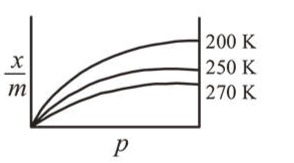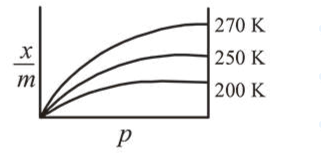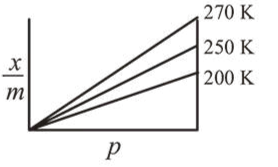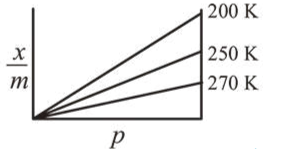Question:

Adsorption of a gas follows Freundlich adsorption isotherm. If $x$ is the mass of the gas adsorbed on mass $m$ of the adsorbent, the correct plot of $\frac{x}{m}$ versus $p$ is :

1.2.3.4.Correct Option: 1

Solution:

As adsorption is exothermic process, hence $\frac{x}{m}$ decreases with increase of temperature.